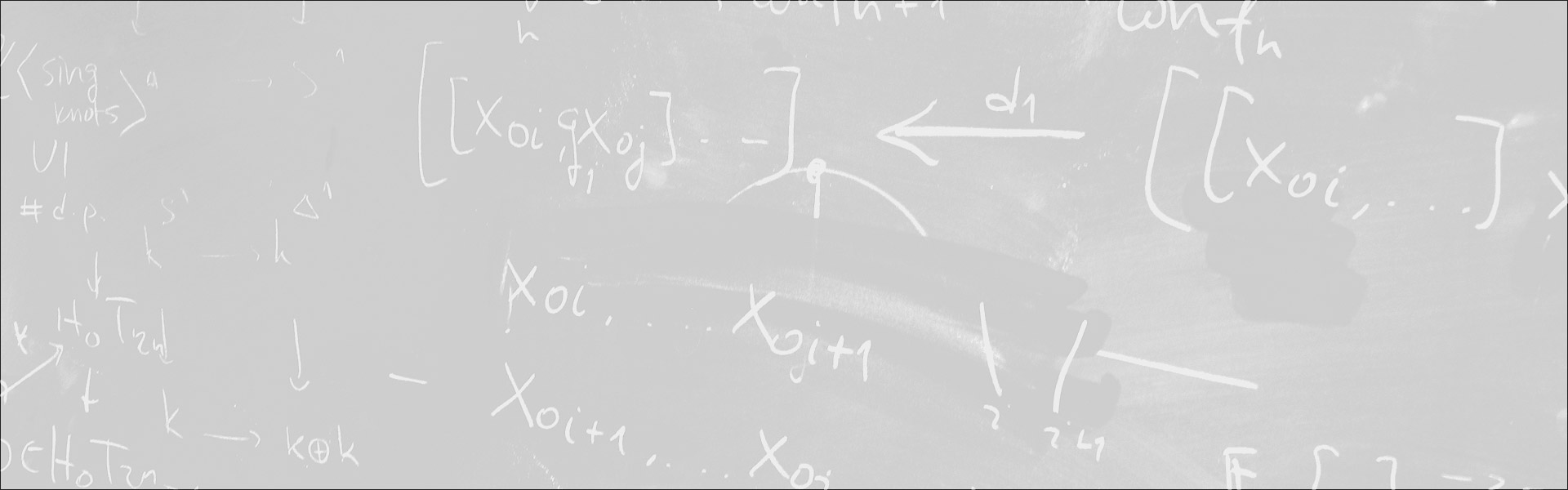Designed and built with care, filled with creative elements

Top# Solving equations in finite groups and complete amalgamation

/  Évènements• Cet évènement est passé

## Solving equations in finite groups and complete amalgamation

Roth’s theorem on arithmetic progression states that a subset A of the natural numbers of positive upper density contains an arithmetic progression of length 3, that is, the equation x+z=2y has a solution in A.Finitary versions of Roth’s theorem study subsets A of {0, … , N}, and ask whether the same holds for sufficiently large N, for a fixed lower bound on the density. In a similar way, concerning finite groups, one may study whether or not sufficiently large sets of a finite group contain solutions of an equation, or even a system of equations. For instance, for the equation xy=z, Gowers (2008) showed that any subset of a finite simple non-abelian group will contain many solutions to this equation, provided it has sufficiently large density.
We will report on recent work with Amador Martin-Pizarro on how to find solutions to the above equations in the context of pseudo-finite groups, using techniques from model theory which resonate with (a group version of) the independence theorem in simple theories due to Pillay, Scanlon and Wagner. In this talk, we will not discuss the technical aspects of the proof, but present the main ideas to a general audience.

- Séminaire Géométrie et théorie des modèles

#### Détails :

Orateur / Oratrice : Daniel Palacin
Date : 15 janvier 2021
Horaire : 11h10 - 12h30
Lieu : Zoom